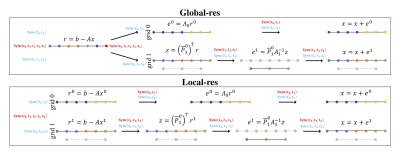# Asynchronous Methods for HPCGlobal-res and local-res partitionings for the Multadd example presented in Section IV for each step of the computation of the corrections e0 ande1. Arrows denote moving to the next step of the computation. Sync() denotes a synchronization point, where the list of threads passed to Sync() denotes the threads that synchronize. Blue Sync() denotes a synchronization for asynchronous multigrid, and red Sync() denotes a synchronization point for synchronous multigrid. Colored points denote points used in a calculation, where t0 is assigned the purple points, t1 is assigned the yellow points, t2 is assigned the blue points, t3 is assigned the orange points, and t4 is assigned the green points. Gray points denote points not used in a calculation.